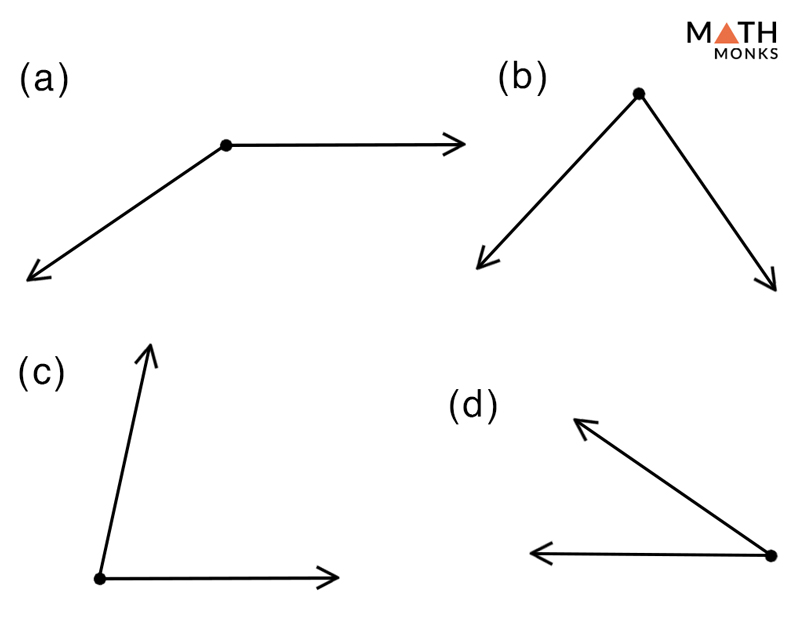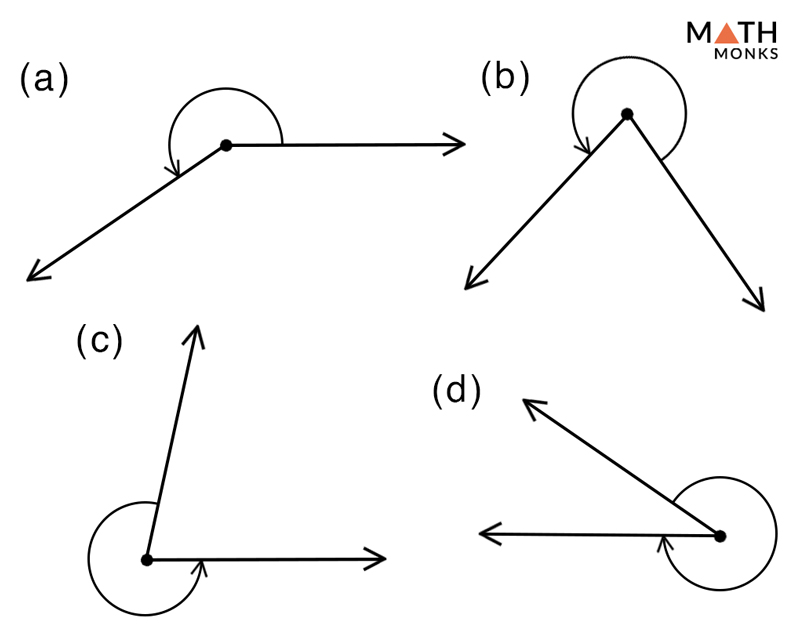# Reflex Angle

## What is a Reflex Angle

A reflex angle is an angle that measures more than 180° and less than 360°. In other words, a reflex angle is equal to the sum of 180° and any of the other primary angles such as acute, obtuse, or right angle.

Thus, for every acute or obtuse angle, there is a reflex angle. Mathematically, the relation is given as,

• Reflex angle = 180° + Acute angle
• Reflex angle = 180° + Obtuse angle
• Reflex angle = 180° + Right angle

A few more examples of reflex angles are given below:

## Reflex Angles in Real Life

The outside angle of anything that makes an acute, obtuse or a right angle such as:

• Outside angles in a triangle, square, rectangle, trapezoid, pentagon, and hexagon
• The letter A or V
• A sliced pizza
• Hands of a clock
• A partially opened compassIdentify and mark the reflex angles in the given shapes.Solution:

The reflex angles in the given options (a), (b), (c), and (d) are shown alongside